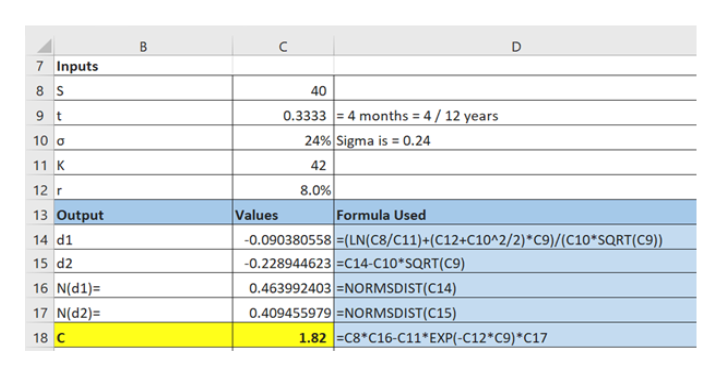# The prices of a certain security follow a geometric Brownian motion with parameters mu=.12 and sigma=.24. If the security's price is presently 40, what is the probability that a call option, having four months until its expiration time and with a strike price of K=42, will be exercised? (A security whose price at the time of expiration of a call option is above the strike price is said to finish in the money.)If the interest rate is 8%, what is the risk-neutral valuation of the call option?

Question
74 views

The prices of a certain security follow a geometric Brownian motion with parameters mu=.12 and sigma=.24. If the security's price is presently 40, what is the probability that a call option, having four months until its expiration time and with a strike price of K=42, will be exercised? (A security whose price at the time of expiration of a call option is above the strike price is said to finish in the money.)

If the interest rate is 8%, what is the risk-neutral valuation of the call option?

check_circle

Step 1

The question has two parts:

• The probability that a call option will get exercised in 4 months - this question has been addressed in the previous question posted by you
• The risk neutral valuation of the call option - I will address this part here.
Step 2

We will evaluate the risk neutral valuation of the call option using the Black Scholes Model.

Inputs are:

• Current stock Price, S
• Strike Price, K
• Risk free rate, r
• Volatility, σ
• Time to maturity, t

The Black Schole formula to value the European call option on a non dividend paying stock is as shown on the white board.

Step 3

Since the entire calculation is intensive, i will resort to excel.

Please see the table on the white board. Please be guided by the last column titled “Formula used” to understand the mathematics. The last r...help_outlineImage TranscriptioncloseC D 7 Inputs 8 S 40 0.3333 = 4 months = 4 / 12 years 24% Sigma is = 0.24 9 t 10 a 11 K 42 8.0% Formula Used 12 r Values 13 Output -0.090380558 (LN(C8/C11)+(C12+C10^2/2)*C9)/(C10*SQRT(C9)) -0.228944623 =C14-C10*SQRT(C9) 14 d1 15 d2 0.463992403 =NORMSDIST(C14) 0.409455979 NORMSDIST(C15) 1.82 C8*C16-C11*EXP(-C12*C9)*c17 16 N(d1)= 17 N(d2)= 18 C fullscreen

### Want to see the full answer?

See Solution

#### Want to see this answer and more?

Solutions are written by subject experts who are available 24/7. Questions are typically answered within 1 hour.*

See Solution
*Response times may vary by subject and question.
Tagged in